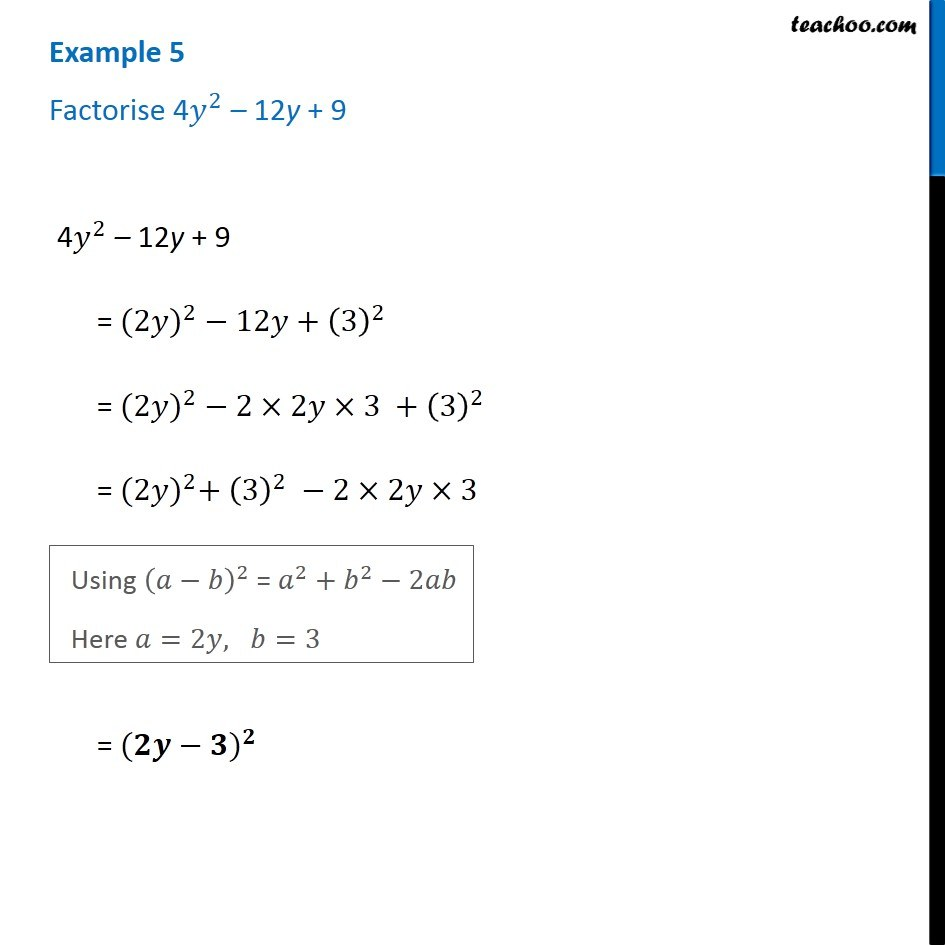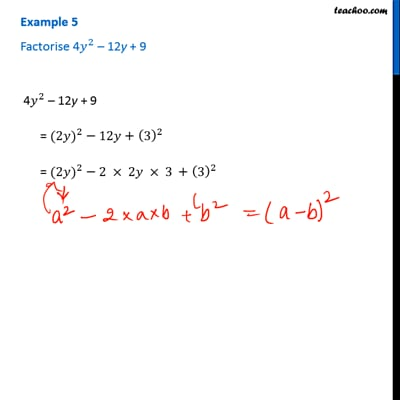Examples

Chapter 14 Class 8 Factorisation
Serial order wiseThis video is only available for Teachoo black users

Get live Maths 1-on-1 Classs - Class 6 to 12

### Transcript

Example 5 Factorise 4𝑦^2 – 12y + 9 4𝑦^2 – 12y + 9 = 〖(2𝑦)〗^2 − 12𝑦+(3)^2 = 〖(2𝑦)〗^2 − 2×2𝑦×3 +(3)^2 = 〖(2𝑦)〗^2+ (3)^2 −2×2𝑦×3 Using (𝑎−𝑏)^2 = 𝑎^2+𝑏^2−2𝑎𝑏 Here 𝑎=2𝑦, 𝑏=3 = (〖𝟐𝒚−𝟑)〗^𝟐